Courses

# Fluid Pressure And Measurement

## 10 Questions MCQ Test Mock Test Series for Civil Engineering (CE) GATE 2020 | Fluid Pressure And Measurement

Description
This mock test of Fluid Pressure And Measurement for Civil Engineering (CE) helps you for every Civil Engineering (CE) entrance exam. This contains 10 Multiple Choice Questions for Civil Engineering (CE) Fluid Pressure And Measurement (mcq) to study with solutions a complete question bank. The solved questions answers in this Fluid Pressure And Measurement quiz give you a good mix of easy questions and tough questions. Civil Engineering (CE) students definitely take this Fluid Pressure And Measurement exercise for a better result in the exam. You can find other Fluid Pressure And Measurement extra questions, long questions & short questions for Civil Engineering (CE) on EduRev as well by searching above.
QUESTION: 1

Solution:
QUESTION: 2

### In a mercury differential manometer used for measuring pressure differences across a venturimeter in a water pipeline, if an error of 2 mm has been made in observing a differential head of 10 mm, the percentage error in pressure difference is:

Solution: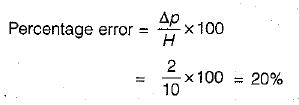QUESTION: 3

### In a water pipeline, the pressure at the highest elevation is a suction pressure of magnitude 4.9 x 102 N/m2. A mercury barometer installed at the place recorded an atmospheric pressure of 98,100 N/m2. The absolute pressure at the highest point of the pipeline is

Solution:
QUESTION: 4

The left leg of a U-tube mercury manometer is connected to a pipeline conveying water, the level of mercury in the leg being 0.6 m below the centre of pipeline, and the right leg is open to atmosphere. The level of mercury in the right leg is 0.45 m above that in the left leg and the space above mercury in the right leg contains Benzene (specific gravity 0.88) to a height of 0.3 m. Find the pressure in the pipe in metres of water.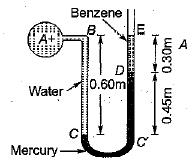Solution: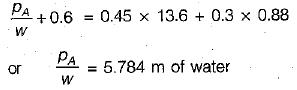QUESTION: 5

The pressure, in meters of oil (sp. gr, 0,8), equivalent to 80 m of water is

Solution: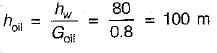QUESTION: 6

Match List-1 (Features) with List-ll .(Type of manometers) and select the correct answer using the codes given below the lists:
List-I
A. Open ended manometer for positive pressure
B. Negative pressure manometer
C. For measuring pressure in liquids or gases
D. For measuring pressure in liquids only

List-ll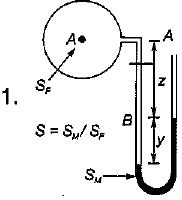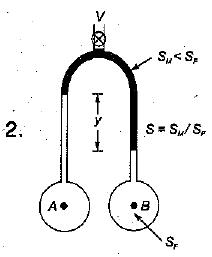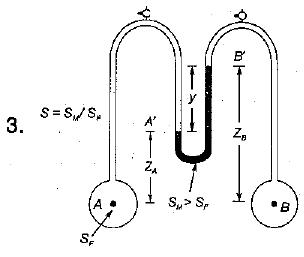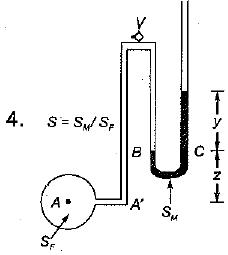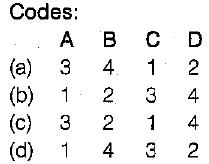Solution:
QUESTION: 7

Absolute pressure in flow system

Solution:

The pressure measured above the absolute zero or complete vacuum is called absolute pressure.
Absolute pressure = Gauge pressure + Local atmosphere pressure.
also Absolute pressure = Local atmosphere pressure - Negative gauge pressure.

QUESTION: 8

Pressure of 200 kPa is equivalent to a head of h metres of carbon tetrachloride of relative density 1.59 where h is equal to

Solution: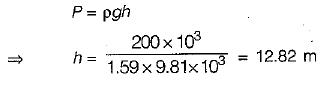QUESTION: 9

Mercury is considered as a superior barometric fluid chiefly due to its

Solution:

Mercury is considered as a superior barometric fluid because of following reasons:
1. High specific gravity- For a same pressure head mercury tube length required will be smaller than that of water
2.Low Vapour Pressure: Thus, influence in readings due to mercury vapours is negligible.

QUESTION: 10

If a Mohr's circle is drawn for a fluid element inside a fluid body at rest, it would be

Solution: